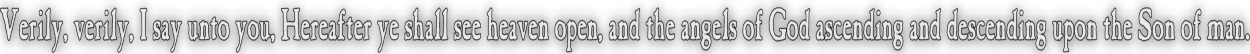None:
Polyps:
Strongs:MetaphysicsIntercession In The Heavens Returning to our trinity model for a moment, we asserted that it is a positive statement or pos(G~x) if x~G, and that pos(x~G) if G is uniquely the correct God. However we have three beings in "heavenly places", G, H and U. We will consider that cogG(H&p) => cogU(H&p) => cogH(H&p) Now we consider that "H~x" is a positive statement So we would infer that it is good for God "to save" x. i.e. cogG(H&x) => cogU(H&x) => cogH(H&x) That we must have pos(H&x) when we also have H~x universally must indicate that in a manner alike to virtues "q" over which we ascribe positive properties "p", we may assume that the intersetion of G and H contains all such x. Then if' 'x' is found in some fashion to be holding ¬L(H) then x is excluded to G \ U. (i.e. G may intercede via U to H to return 'x' to L(G) upon repentance, and back into agreement with U i.e. that pos(H&x). Then we will exclude from G also all those that are not redeemed (by repentance). So H~x universally. G~x if x is redeemed, U~x if x is saved. We have that U~x <=> x holds fast L(G) G~x if x holds ¬L(G) but only temporarily H~x universally, but pos(H~x) <=> G~x. Then if U is the strictest form of containment, we ascribe such x the quality of "virtue", and those in G \ U the quality of positive properties, and those in H not in G those "negative" statements "¬pos(p)" We find we may immediately make some sense of U = "G ∩ H" (Then pos(U~x) if both pos(H~x) and pos(G~x)) Or with our model 0 = [a,b,c,d,e,f,g] = all x. (everything H~x) a = 1 = [a,b,c] = {q} the saved, pos(U~x) x holds L(G) intact b = [a,d,e] = {q},{p1,p2,p3} the redeemed, pos(G~x) x holds L(G) "almost everywhere." c = [a,f,g] = {q}, {q1,q2,q3} those once holding L(G) but now holding fast to ¬L(G). Then we see that in the octal we must include all those whom never held L(G) in H \ G. Then in all accord to our model, the "saved" in U are equivalent to the unity element in the K4 ultrafilter, and those whom the Lord never knew are those in the Father (octal) without Christ. The ones of whom he states "Away from me, ye workers of iniquity" are those whom have passed out as those "qi" privated by the grafting on of those "pi" correctly holding L(G). They as "qi" are put to shame as if they never held L(G). where "pi" are those to whom he refers as His "these" in "When you did not do it for the least of these, ye did it not unto me." Then we have our intercession: for if a believer holds to a G v p statement and violates but temporarily L(G) - then He is displaced from U into G \U. then by virtue of intercession G may intercede as to; pos(G&x) which asserts; by G relating to H by reason of U (NB: G is as a "subset" of H.) pos(cogG(H&x)) => pos(cogU(H&x)) => pos(cogH(H&x)) => pos(H&x) => H&x then pos(G~x) and pos(H~x) => pos((G ∩ H) ~x) => pos(U&x) but if pos(U&x) then x has returned to L(G). i.e. x repented. And we have merely treated x as any other positive property of G and H. Continue To Next Page Return To Section Start Return To Previous Page'